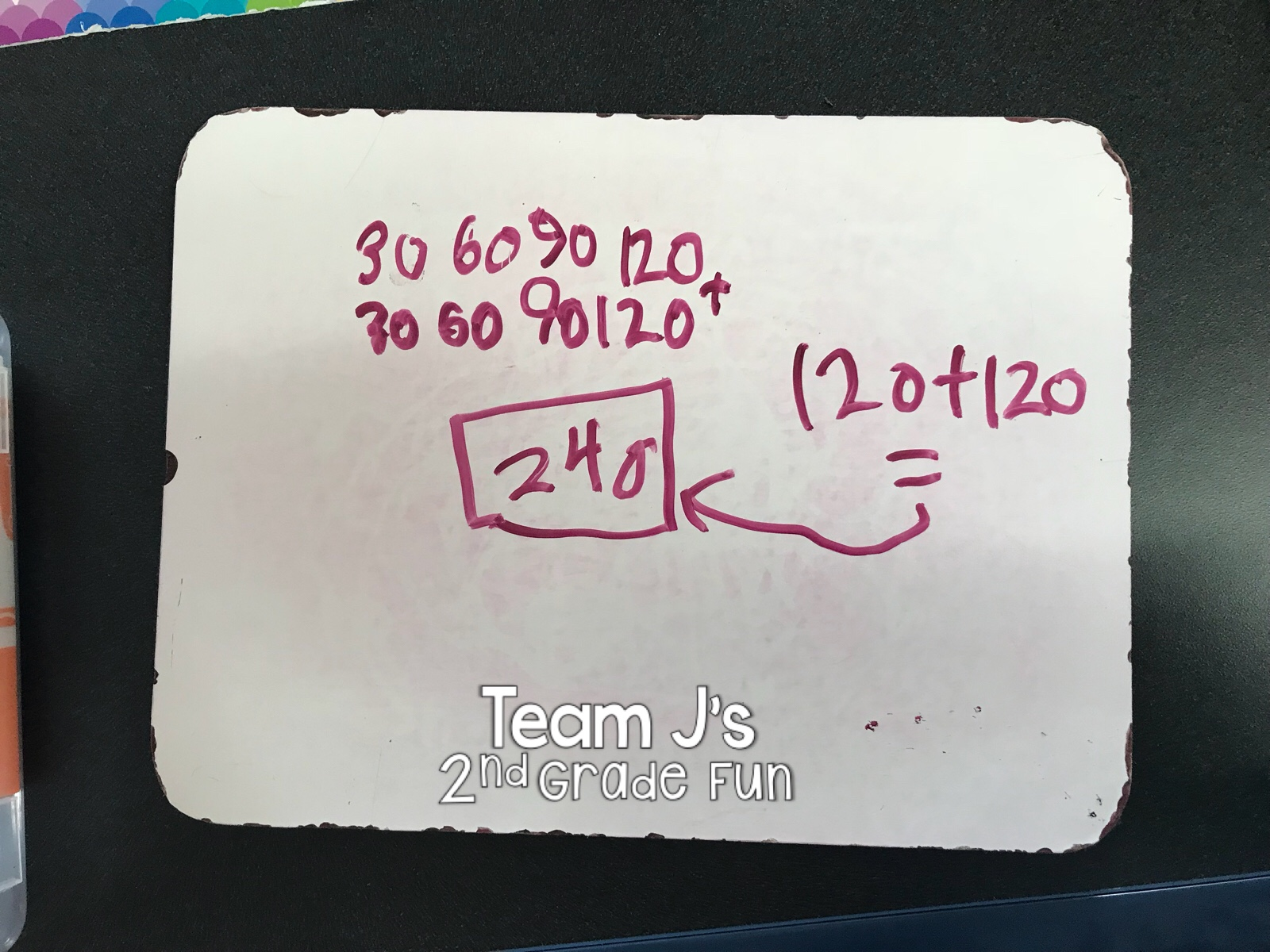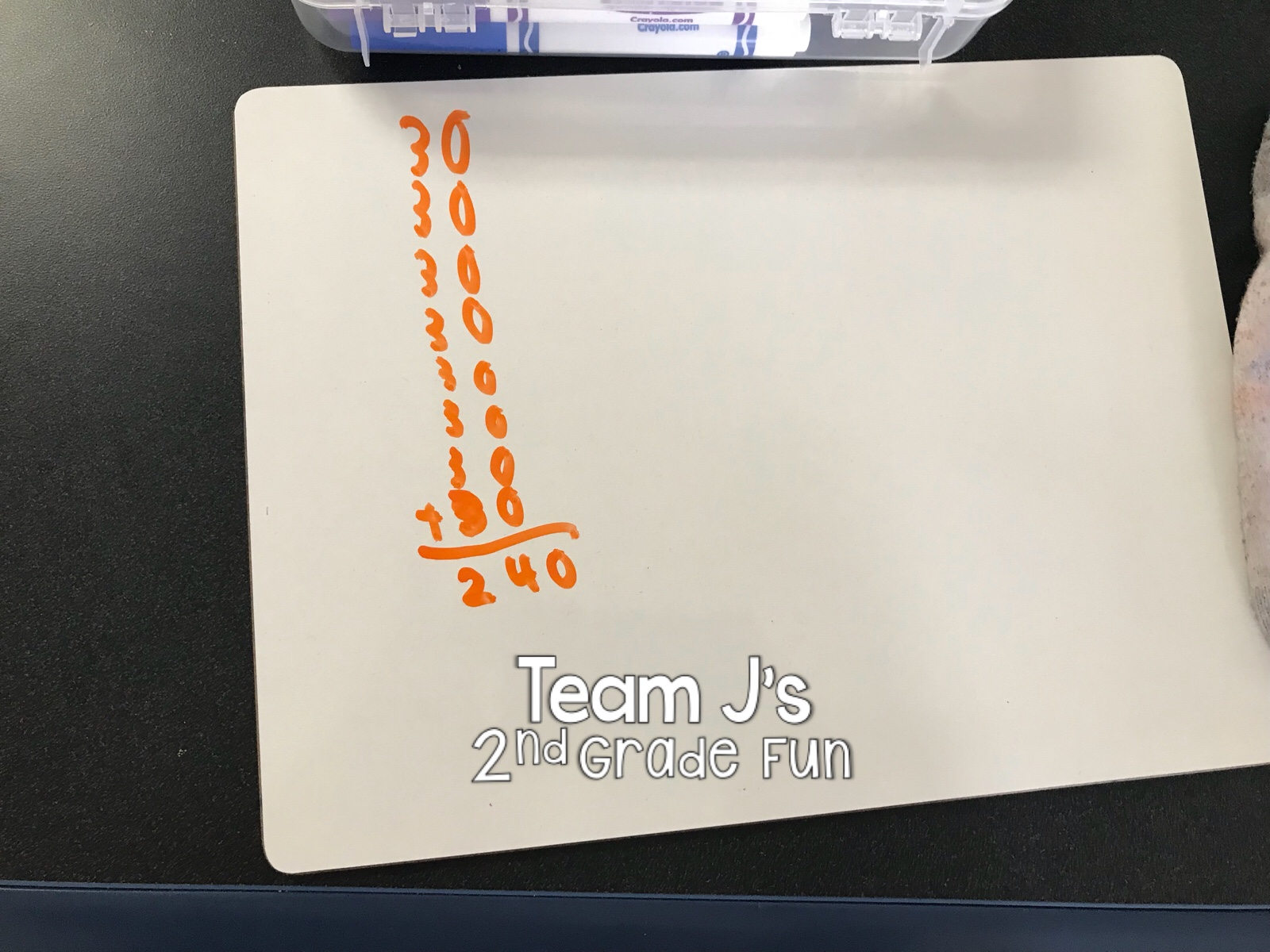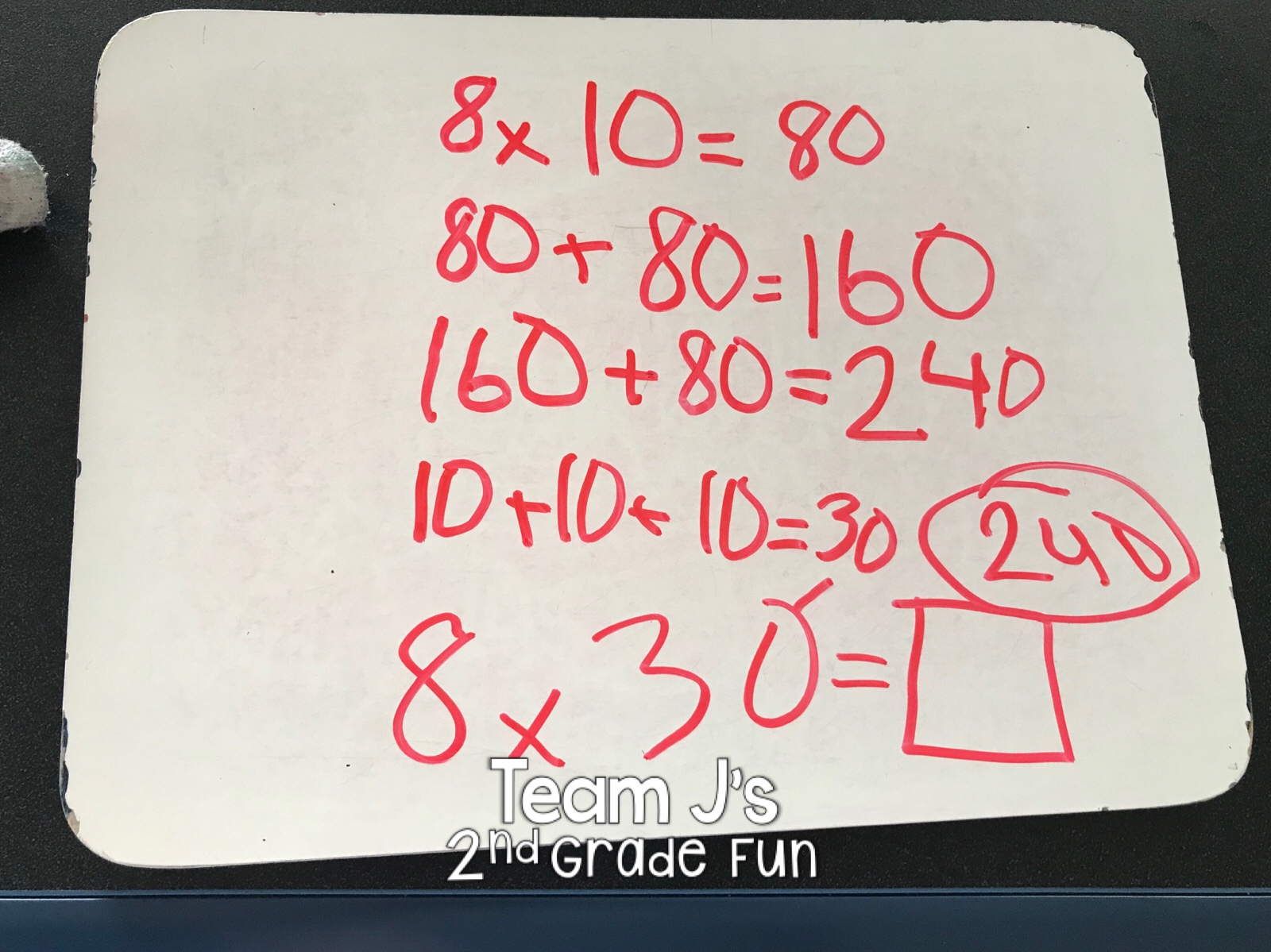Browsing Tag

# Math Strategies

Math has always been one of my favorite subjects to teach.  I think one of the main reasons why I enjoy teaching math so much is the variety of strategies that can be used to solve a math problem.  After reading this post you’ll learn four different subtraction math strategies that your students could use to help boost their understanding and math problem solving abilities.

## Subtraction Math Strategies:

Below you will see four different subtraction math strategies that your students might use.  Each student will be in a different spot with their math strategies. For example, some students need the concrete/manipulatives and place value strategy will be best for them.  Other students might be really good at breaking numbers apart and using them in different ways – then break apart or expanded might be best for them.  With math strategies there is not a one size fits all type of mentality.  Students can use a variety of strategies and will move through them at different rates when they are ready.

### Place Value Strategy:

Draw out how many there are to start with – 95  Then, take away or cross out how many are being taken away – 56.  Start by crossing out the 5 tens for 50.  Then, you have 5 ones and you can’t take away 6 from 5 so, take a ten and regroup it into 10 ones.  (Circle the ten and change it into the ten ones (dots)).  Now, you can take away the 6 ones.  Then, count what is left – 10, 20, 30, and 9 more so 39.

### Empty Number Line Strategy:

First, draw an empty number line.  Then, start at the number that you’re beginning with – 95.  95 will go on the right side of the empty number line because you are subtracting so your number will be getting smaller.  Then, 56 can be taken away a few different ways. In this example, they first took away 50 and got to 45. Then, took away 5 and got to 40 (friendly number) and one more and got to 39.  Students could also take away all 6 ones.  The 50 could also be broken down into – 20, 20, 10 – 10, 10, 10, 10, 10 – 30, 20 – 40, 10 – etc.

### Break Apart Strategy:

For break apart, leave the first number together since this is the number that you are starting with.  Then, break apart the number that you are taking away.  So, 56 would break into 50 and 6 (expanded notation).  Then, 95 – 50 = 45 and 45 – 6 = 39.

### Expanded Form Strategy:

First, change each number into expanded notation. 95 = 90 + 5 and 56 = 50 + 6.  Then, subtract the ones from the ones.  You can’t take away 6 from 5.  So, you take ten from the 90 to add to the ones.  When you take ten from the 90, the 90 turns into 80.  Then, add the ten you took to your ones 10 + 5 = 15.  Now, you can subtract.  15 – 6 = 9 and 80 – 50 = 30.  Then put the numbers back together 30 + 9 = 39.

## Subtraction Practice:

If you’re looking for some subtraction practice pages for your students to practice these strategies – I have worksheets already created for you.  Best part is – they are differentiated into 3 levels! These Subtraction Word Problem Printables are great for independent practice, homework, formative assessments, and more. Check them out here >>> Subtraction Differentiated Word Problem Worksheets.

If you are interested in learning about Addition Math Strategies, check out my blog post>>>HERE.

Math has always been one of my favorite subjects to teach.  I think one of the main reasons why I enjoy teaching math so much is the variety of strategies that can be used to solve a math problem.  After reading this post you’ll learn four different addition math strategies that your students could use to help boost their understanding and math problem solving abilities.

## Variety of Math Strategies:

While in math there is typically one right answer – there are many different ways you can get there. When I was in school I was good at math and got the right answer, but had no idea how or why.  For addition, my teacher taught me one way – with carrying over and I did and I got the answer. But, I didn’t really understand the “why” behind the math. What I love about teaching math now is we let kids figure it out for themselves.  We give kids a chance to explore multiple strategies and find the right one for them. There is no more one size fits all.  I wish I had learned math this way when I was younger (and please know I’m not faulting my past teachers – this is just the way it was taught then).

Below you will see four different addition math strategies that your students might use.  Each student will be in a different spot with their math strategies. For example, some students need the concrete/manipulatives and place value strategy will be best for them.  Other students might be really good at breaking numbers apart and using them in different ways – then break apart or expanded might be best for them.

Students can also move through the strategies.  One student might start with place value strategy, but as they become more comfortable in math they then move on to empty number line or one of the others. Students aren’t pigeon holed into their strategy. Think of it almost like a buffet where they can try the different strategies and see which one works best for them.

### Place Value Strategy:

Start by drawing out the place value model using base 10 blocks for each number.  Students would draw 3 tens sticks and 9 ones dots to represent 39 and then below 2 tens sticks and 8 ones dots to represent 28.  Then, count the ones.  There are 17 ones.  Since there are more than 10, you would regroup.  Circle the ten ones and draw an arrow to the new ten that you made over in the tens area.  Then count up your tens (10, 20, 30, 40, 50, 60) and 7 ones and your answer will be 67.

### Empty Number Line Strategy:

For empty number line strategy you start with an empty line.  Students have a choice and can either start at 39 or 28.  This is a great learning opportunity to talk about what is more efficient.  It would be easier to count up 28 then 39.  So on the left side of the number line, place the number 39.  Then, for counting there are multiple options.  We want to avoid students counting all 28 by ones so they can break it into tens and ones. Some students might start at 39 and jump 10 to 49 and 10 to 59 and then count up 8 ones to get to 67. Some students might start at 39 and jump 20 to 59 and then 8 ones to get to 67. There are multiple ways students can use the number line to help them solve the problem.

### Break Apart Strategy:

For the break apart strategy it is just what it sounds like – students are going to break the numbers apart into tens and ones. 39 breaks into 30 and 9. 28 breaks into 20 and 8.  Students will then add 30 and 20 and get 50. Then, add 9 and 8 and get 17. Then they’ll add 50 and 17 to get 67.

### Expanded Form Strategy:

This is one of my favorite strategies and it is very similar to the break apart strategy.  Students will start by writing both numbers in expanded form.  39 expands to 30 + 9 and 28 expands to 20 + 8. These numbers will be written vertically on top of each other (ones on top of ones, tens on top of tens).  Then students add straight down.  9 + 8 = 17. The student will write down 7 in the ones spot and then move the 10 up to the 10s spot.  Then add 10 + 30 + 20 = 60.  Then, put the numbers back together 60 + 7 = 67.

If you’re looking for some addition practice pages for your students to practice these strategies – I have worksheets already created for you.  Best part is – they are differentiated into 3 levels! These Addition Word Problem Printables are great for independent practice, homework, formative assessments, and more. Check them out here >>> Addition Differentiated Word Problem Worksheets.

One of the skills we cover in second grade (which I know is often a third grade skill) is multiplying by multiples of 10. This can off scare kids as you are using larger numbers, but I knew my kids could handle it. To make it a little less scary, I introduced it using a story problem. I put the problem below up on the board and read over it with the kids.Then, I told the kids to solve it on their white boards at their seats. I didn’t give any prompting or suggestions, I wanted to see what they would come up with on their own.  Boy was I pleasantly surprised! They had amazing strategies! As you’ll see below they came up with multiple different ways to come to the answer.  They all understood it was equal groups and they used strategies we had talked about with multiplication – drawing out equal groups, skip counting, repeated addition, breaking apart numbers, etc. I was so proud of them. After giving them time to solve I had students bring their white board up to explain their strategies to the class.

This honestly was the best way I have ever introduced it. Instead of me telling them how to figure it out or only showing them the trick (8 x 3 = 24 so 8 x 30 = 240), they really took them time to try to figure it out for themselves. And it helped because on future problems they knew multiple strategies they could use to solve it.

See their awesome strategies below…